The sum graphically

Draw a graphically sum of the all sides of 4-gon ABCD.

Leave us a comment of example and its solution (i.e. if it is still somewhat unclear...):Be the first to comment!Next similar examples:

1. RectangleDraw a rectangle with the sides a = 4 cm, b = 5 cm. Mark the center of symmetry S and all axes of symmetry. How many axes of symmetry has? Write down.
2. Square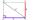Draw a square on the edge of a = 4 cm. Mark the center of symmetry S and all axes of symmetry. How many axes of symmetry does? Write down.
3. Three points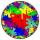Mark three points E, F and G in the plane not lie on one line. a) Draw a line segment FG b) Construct halfline (ray) EG c) Draw a line EF
4. Straight lines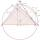Draw two lines c, d so that c || d. On line c mark points A, B, from point A start perpendicular to line c, from point B perpendicular to line c.
5. Rhombus sidesfind the sides of a rhombus, it area is 550 cm square and altitude is 55cm
6. Strip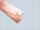From 5.9 cm wide strip should be cut rhombus with area 28 cm2. How long will be its side?
7. RhombusFind the length of each side of rhombus if the perimeter is 49 dm long.
8. TriangleProve whether you can construct a triangle ABC, if a=9 cm, b=10 cm, c=4 cm.
9. DoctorsIn the city operates 171 doctors. The city has 128934 citizens. How many citizens are per one doctor?
10. Roman numerals 2+Add up the number writtens in Roman numerals. Write the results as a roman numbers.
11. Train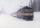The train passes part of the line for 95 minutes at speed 75 km/h. What speed would have to go in order to shorten the driving time of 20 minutes?
12. Compare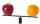Compare with characters >, <, =: 85.57 ? 80.83
13. Roman numerals +Add up the number writtens in Roman numerals. Write the results as a decimal number.
14. To thousands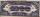Round to thousands following numbers:
15. ProductResult of the product of the numbers 1, 2, 3, 1, 2, 0 is:Added together and write as decimal number: LXVII + MLXIVWrite numbers written in Roman numerals as decimal.